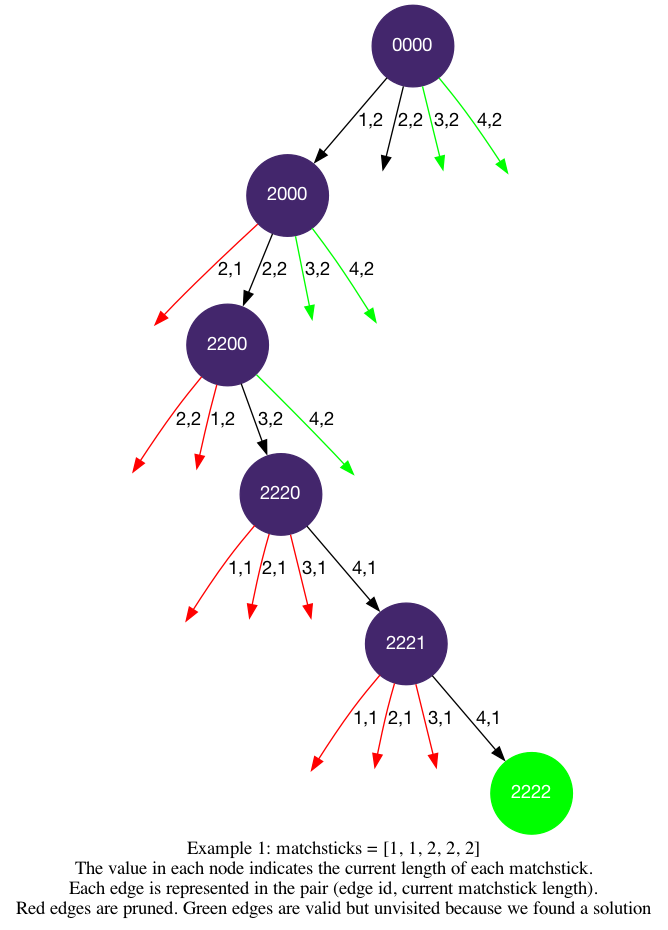# LeetCode Matchsticks to Square Solution

You are given an integer array `matchsticks` where `matchsticks[i]` is the length of the `ith` matchstick. You want to use all the matchsticks to make one square. You should not break any stick, but you can link them up, and each matchstick must be used exactly one time.

Return `true` if you can make this square and `false` otherwise.

Example 1:Input: `matchsticks = [1,1,2,2,2]`

Output: `true`

Explanation: You can form a square with length 2, one side of the square came two sticks with length 1.

Example 2:

Input: `matchsticks = [3,3,3,3,4]`

Output: `false`

Explanation: You cannot find a way to form a square with all the matchsticks.

Constraints:

• `1 <= matchsticks.length <= 15`
• `1 <= matchsticks[i] <= 108`

## Solution

We are looking to build four sides of the same length, and we can easily calculate the target side length by `sum(matchsticks)//4`. Then we can solve this problem by trying to put each matchstick on the four different sides, and see if we can reach a solution.

We can apply the backtracking2 template for aggregation, as we are only asked to find whether a solution exists.

Let's fill in the logic.

• `is_leaf`: `start_index == len(matchsticks)`, when we have used all of the matchsticks.
• `get_edges`: we can put a match stick on four different sides.
• `is_valid`: `sides[i] + matchsticks[start_index] <= side_length`, the choice is valid only when the new `side[i]` length will not exceed `side_length`.
• additinal states: `sides` that stores the current length of the four sides.

#### Implementation

``````1def makesquare(self, matchsticks: List[int]) -> bool:
2    if sum(matchsticks) % 4 != 0: return False
3    side_length = sum(matchsticks) // 4
4    matchsticks.sort(reverse=True)
5    sides = [0, 0, 0, 0]
6    def dfs(start_index):
7        if start_index == len(matchsticks):
8            return side_length == sides == sides == sides == sides
9
10        for i in range(4):
11            if sides[i] + matchsticks[start_index] <= side_length:
12                sides[i] += matchsticks[start_index]
13                if dfs(start_index + 1): return True
14                sides[i] -= matchsticks[start_index]
15        return False
16    return dfs(0)  ``````